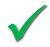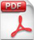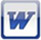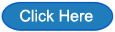Access 2003: Database queries

The ticks indicate the suitability of resources for each Key Stage.

KS5

Introduction to queries

This workbook assumes that student know how to set up a simple one table database in Access.

They are then taken step by step through creating different types of query.

Students can work at their own pace by following the instructions given in the workbook.This task assumes that students are familiar with using an Access database.

They are given a prepared database and asked to find out the answers to a series of questions.cars.mdb

This task assumes that students are familiar with using an Access database.

They are given a prepared database and asked to find out the answers to a series of questions.queries2.mdb

This task provides students with a pre-prepared database.

They are asked to write specific queries using the database.

Assumes some knowledge of Access and writing queries.queries3.mdb